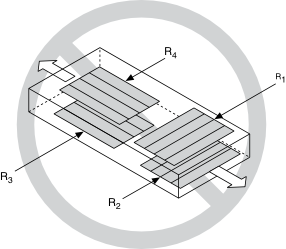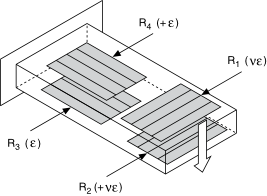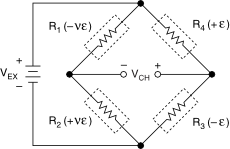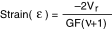# Full-Bridge Type II

## NI-DAQmx 18.6 Help

Edition Date: January 2019
Part Number: 370466AH-01
View Product InfoNI-DAQmx 18.6 HelpNI-DAQmx 19.0 HelpNI-DAQmx 19.1 HelpNI-DAQmx 19.5 Help

The full-bridge type II configuration only measures bending strain.The following figure shows how to position strain gage elements in a bending configuration for the full-bridge type II.Full-bridge type II strain gage configurations have the following characteristics:
• Four active strain gage elements. Two are mounted in the direction of bending strain with one on the top side of the strain specimen and the other on the bottom side. The other two act together as a Poisson gage and are mounted transverse, or perpendicular, to the principal axis of strain with one on the top side of the strain specimen and the other on the bottom side.
• Rejection of axial strain.
• Compensation for temperature.
• Compensation for the aggregate effect on the principle strain measurement due to the Poisson's ratio of the material.
• Sensitivity at 1000 µε is ~ 1.3 mVout / VEX input.
Related concepts

## Full-Bridge Type II Circuit DiagramThe following symbols apply to the circuit diagram:
• R1 is the active strain gage element measuring compressive Poisson effect (–ε).
• R2 is the active strain gage element measuring tensile Poisson effect (+ε).
• R3 is the active strain gage element measuring compressive strain (–ε).
• R4 is the active strain gage element measuring tensile strain (+ε).
• VEX is the excitation voltage.
• RL is the lead resistance.
• VCH is the measured voltage.

The following equation converts voltage ratios to strain units for full-bridge type II configurations.where Vr is the voltage ratio that virtual channels use in the voltage-to-strain conversion equation, GF is the gage factor, and v is the Poisson's ratio.